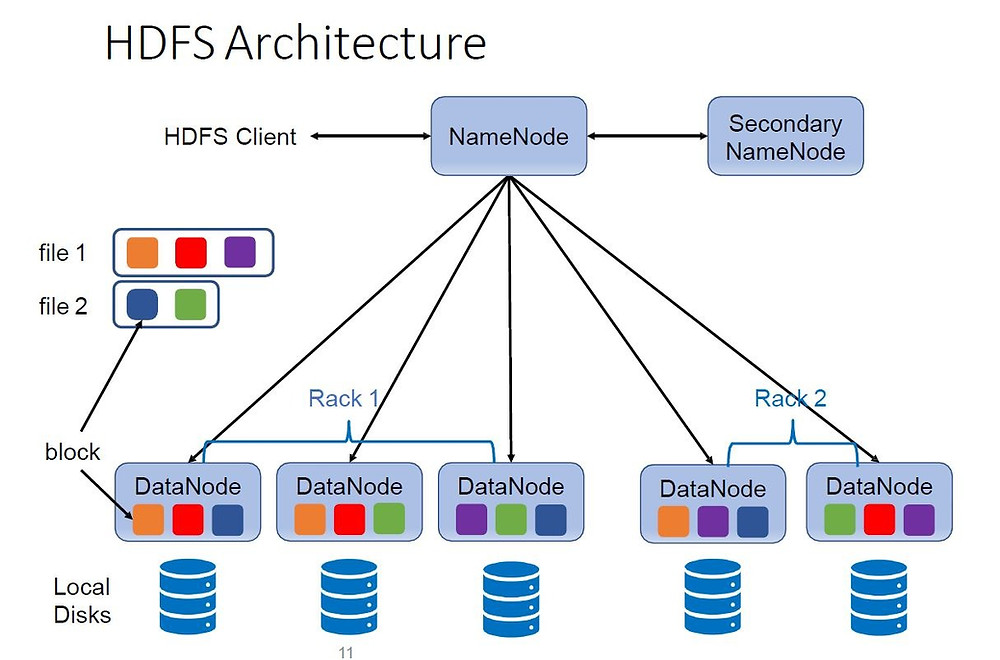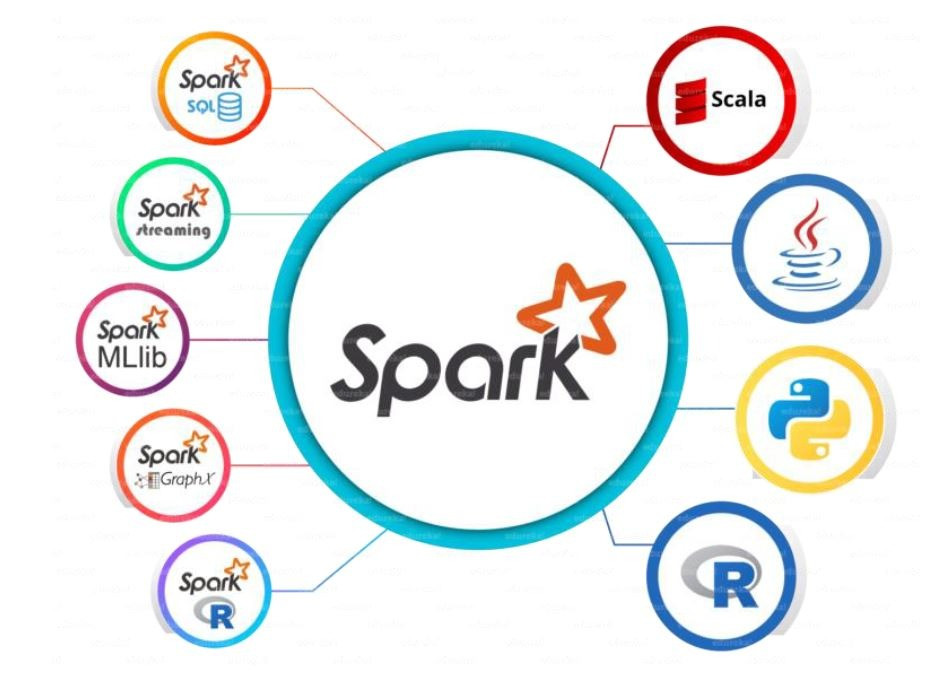Search

# Big Data, HDFS, Spark Project Help

Updated: Mar 25

Hadoop is an open-source framework that allows to store and process big data in a distributed environment across clusters of computers using simple programming models. It is designed to scale up from single servers to thousands of machines, each offering local computation and storage.Q1. HDFS

Let N be the number of DataNodes and R be the total number of blocks in the

DataNodes.

Assume the replication factor is 5, and k out of N DataNodes have failed

simultaneously.

1. Write down the formula of Li(k,N) for i 2 {1, . . . , 5}, where Li(k,N) is the number of blocks that have lost i replicas.

2. Let N = 500, R = 20, 000, 000, and k = 200. Compute the number of blocks that cannot be recovered under this scenario. You need to show both the steps and the final result to get full credit.

Q2. Spark

Consider the following PySpark code snippet:```raw_data = [("Joseph", "Maths", 83), ("Joseph", "Physics", 74),
("Joseph", "Chemistry", 91), ("Joseph", "Biology", 82),
("Jimmy", "Maths", 69), ("Jimmy", "Physics", 62),
("Jimmy", "Chemistry", 97), ("Jimmy", "Biology", 80),
("Tina", "Maths", 78), ("Tina", "Physics", 73),
("Tina", "Chemistry", 68), ("Tina", "Biology", 87),
("Thomas", "Maths", 87), ("Thomas", "Physics", 93),
("Thomas", "Chemistry", 91), ("Thomas", "Biology", 74)]

rdd_1 = sc.parallelize(raw_data)
rdd_2 = rdd_1.map(lambda x:(x, x))
rdd_3 = rdd_2.reduceByKey(lambda x, y:max(x, y))
rdd_4 = rdd_2.reduceByKey(lambda x, y:min(x, y))
rdd_5 = rdd_3.join(rdd_4)
rdd_6 = rdd_5.map(lambda x: (x, x+x))
rdd_6.collect()```

1. Write down the expected output of the above code snippet.

2. List all the stages in the above code snippet.

3. What makes the above implementation inefficient? How would you modify the code and improve the performance?

Q3: LSH

Consider a database of N = 1, 000, 000 images. Each image in the database is pre-processed and represented as a vector o 2 Rd. When a new image comes as a query, it is also processed to form a vector q 2 Rd. We now want to check if there are any duplicates or near-duplicates of q in the database. Specifically, an image o is a near duplicate to q if cos((o, q))  0.9. We want to find any near-duplicate with a probability of no less than 99%.

We now design an LSH scheme using SimHash to generate candidate near duplicates. Assume that for query q, there are 100 images that are near-duplicate to q.

1. Assume k = 5, how many tables does the LSH scheme require (i.e., L) to ensure that we can find any near-duplicate with probability no less than 99%?

2. Consider image o with cos((o, q)) < 0.8, k = 5 and L = 10. What is the maximum value of the probability of o to become a false positive of query q?

You need to show the intermediate steps along with the final result to get full credit.

If you need a solution to these questions or other help related to Big Data, HDFS, SPARK, LSH, etc., then you can contact us at the given link: Codersarts You can send the details of your requirements at: contact@codersarts.com

### Recent Posts

See All

#### Machine Learning With R | Sample Assignment | Assignment Help

Tel: (+91) 0120  4118730

Time :   10 : 00  AM -  08 : 00 PM IST